ISSAC 96 - Hypergeometric Function Representations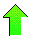Contents News View

 Implementation Specifics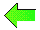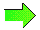The main routine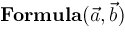computes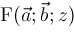.  There is a subroutine Lookup which computes a suitable origin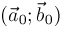for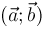.  There is a subroutine Plan which determines the proper sequence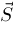of shift operators and inverse shift operators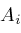,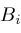,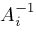, and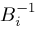which should be applied to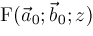to produce.  After the plan is computed, a loop executes the plan by calling subroutines Shift and Unshift.  The Unshift routine calls a routine Contig which computes contiguity relations and also calls Shift

For aesthetic reasons (i.e. pretty answers) all the routines work, until the very last moment, in terms of a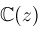-module basis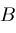.  In fact, in the current implementation,is always (z),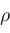is a positive integer, and the answer will be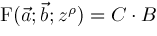after each step of the main loop where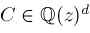is a coefficient vector.  The basisis generally some vector of expressions involving special functions.  The derivative matrix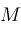which has elements in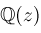satisfies the equation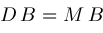where.  At the last moment,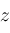is replaced by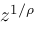since we are interested in computinginstead of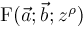.  Often,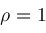, but not always.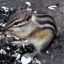©2004-2018 Planet Quantum Kelly Roach